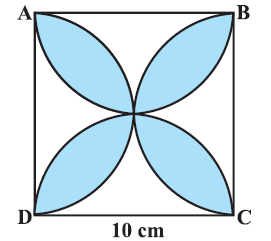# Areas?

Geometry Level 3Find the area of the shaded region, where ABCD is a square of side 10 cm and semi circles are drawn with each side of the square as diameter. Take $\pi = 3.14$ and express your answer in $cm^2$.

×